# RD Sharma Solutions for Class 9 Maths Chapter 9 Triangle and its Angles

## RD Sharma Solutions Class 9 Maths Chapter 9 – Free PDF Download

RD Sharma Class 9 Solutions is provided here for students to clear all their doubts regarding triangles as well as problems based on this topic. The solutions are so apt that students who practice on a regular basis undoubtedly secure high marks in their academics. RD Sharma Solutions Class 9 Chapter 9 – Triangle and its Angles, includes three exercises and all questions are solved by BYJU’S subject experts. In this chapter, students will study a plane figure formed by three non-parallel lines in a plane, called a triangle. A triangle is a 2D geometrical figure consisting of three edges and three vertices.

The three angles are made by the three sides of a triangle and the sum of all the internal angles of a triangle is equal to 180 degrees. Triangles are categorized based on their angles: Acute Triangle, Right Angle and Obtuse Triangle. For a better understanding of concepts, students can download the solutions in PDF whenever required. RD Sharma Solutions are the best material for students who aspire to become proficient in Mathematics. The solutions are designed based on the latest CBSE syllabus as per the student’s intelligence quotient.

## Download PDF of RD Sharma Solutions for Class 9 Maths Chapter 9 Triangle and its Angles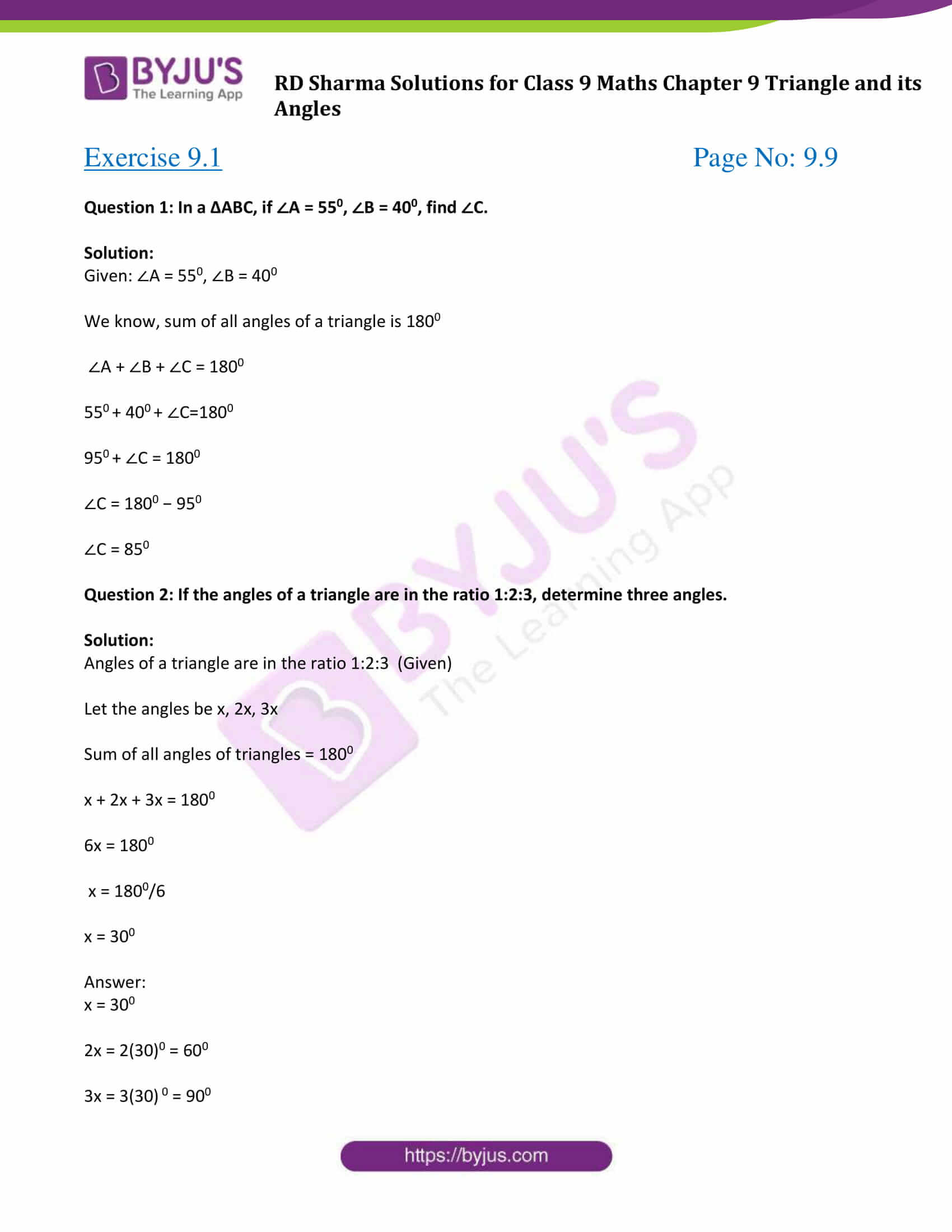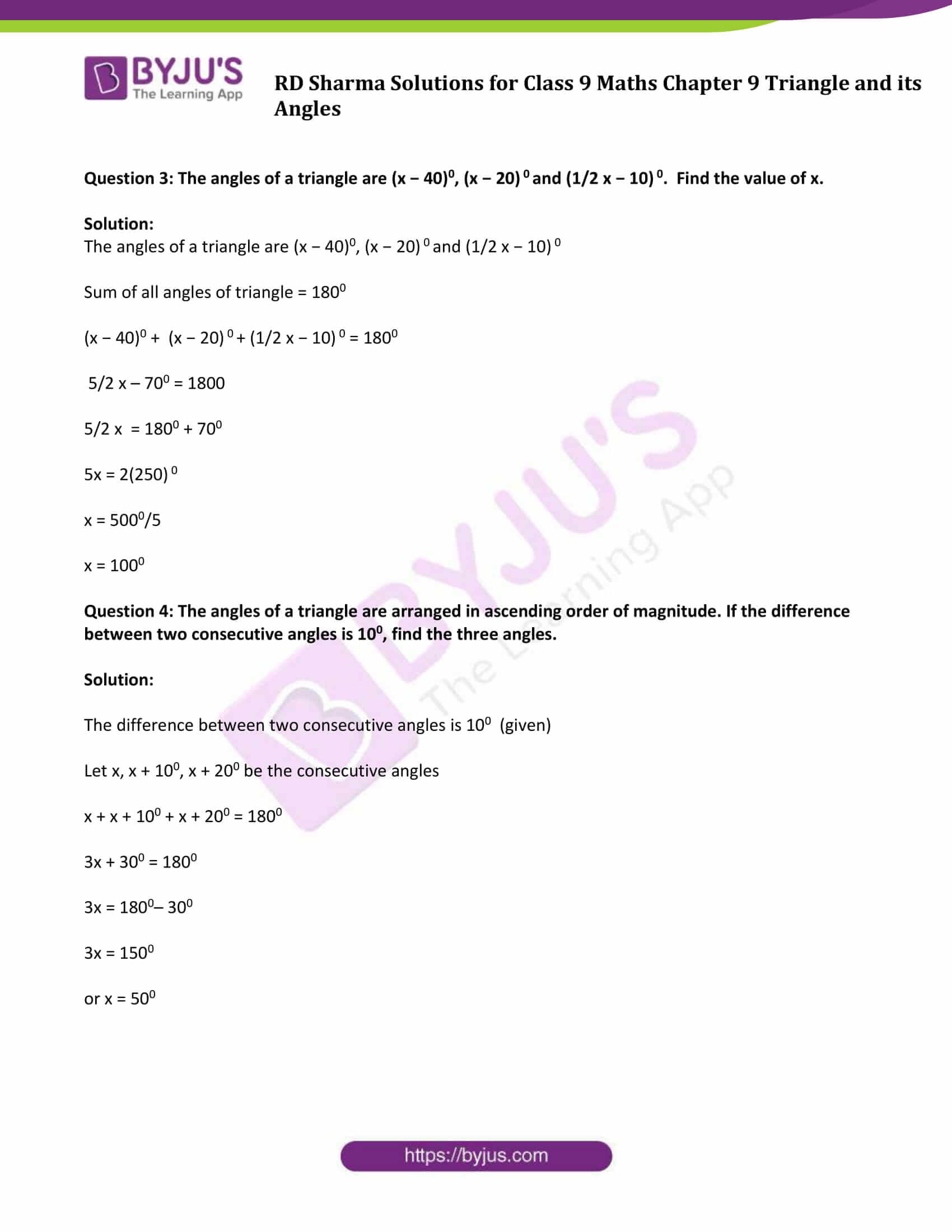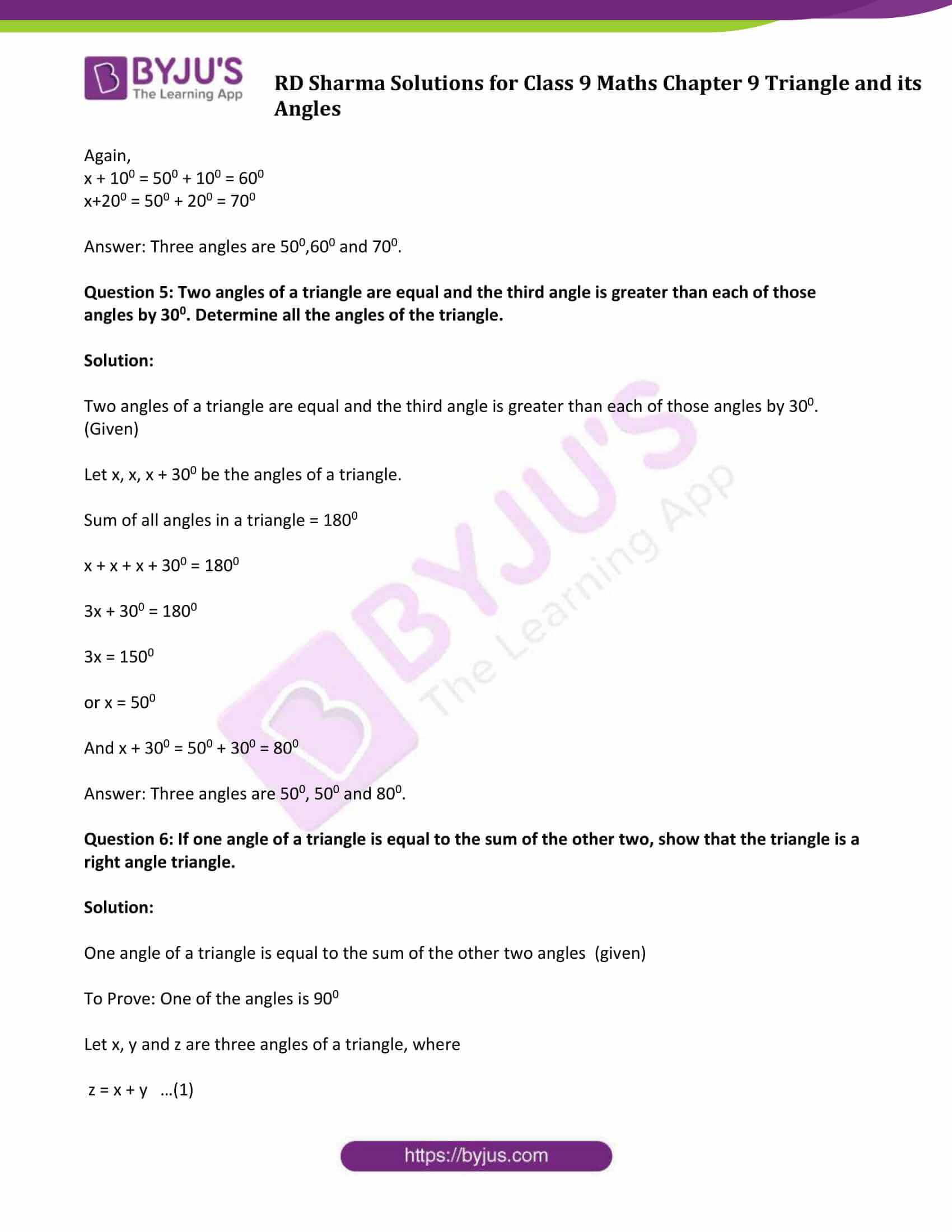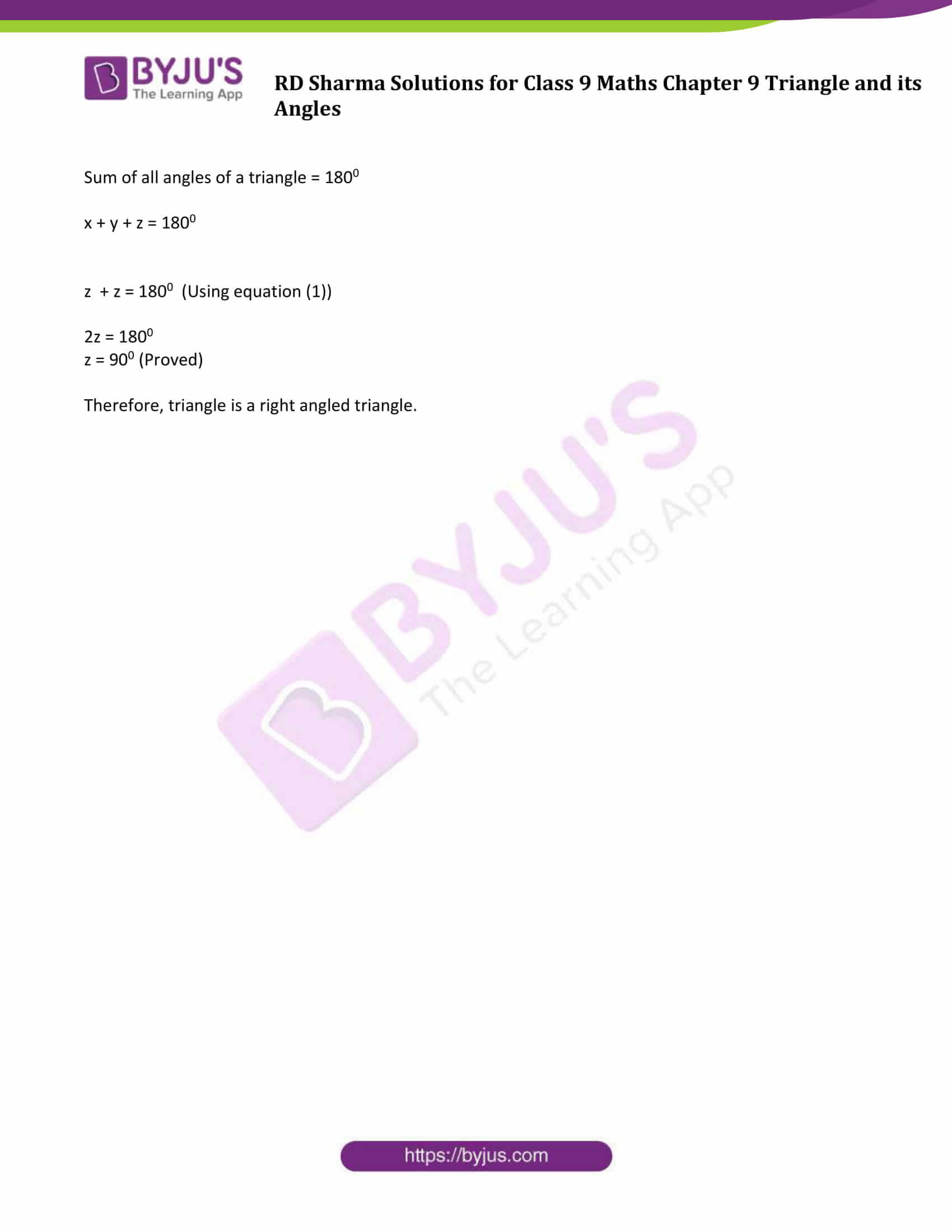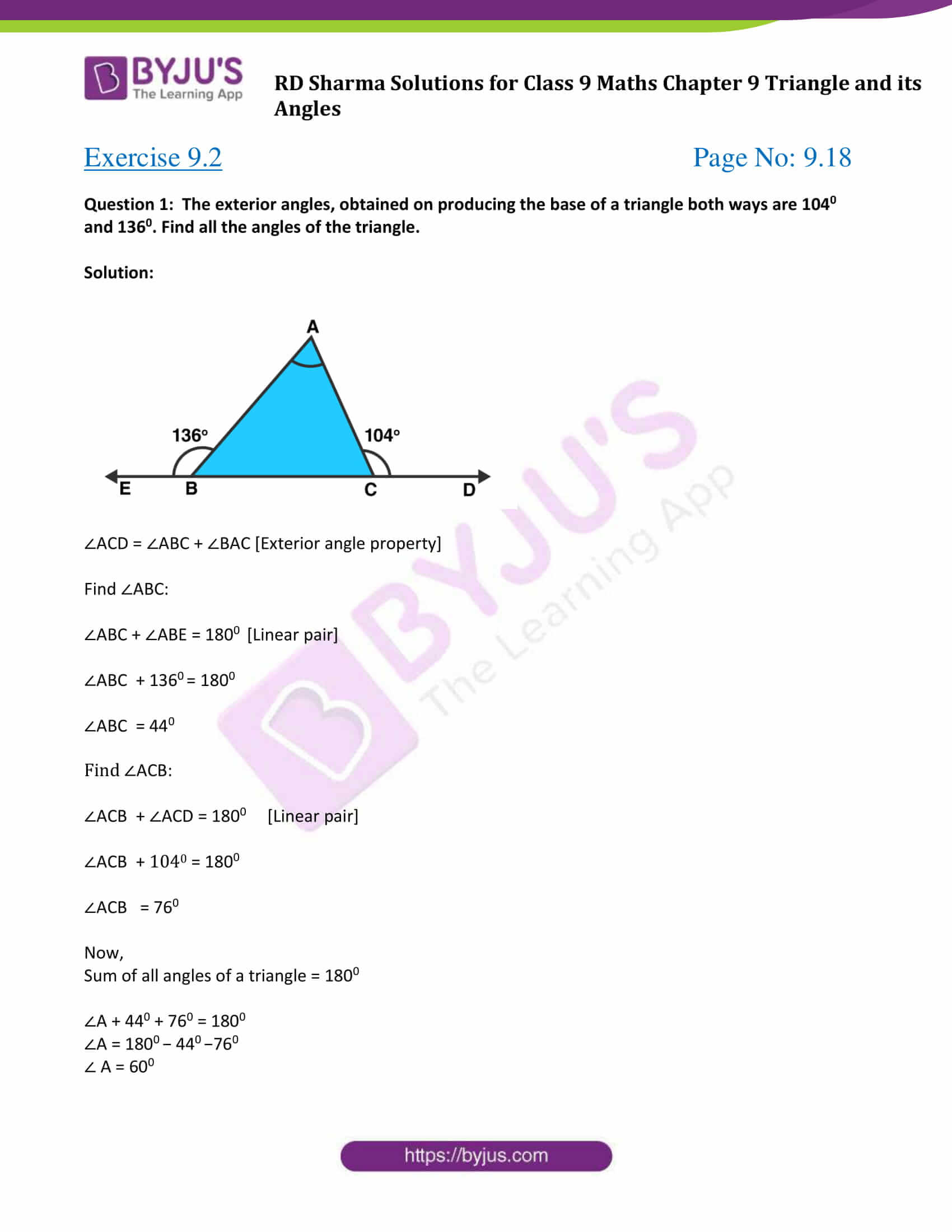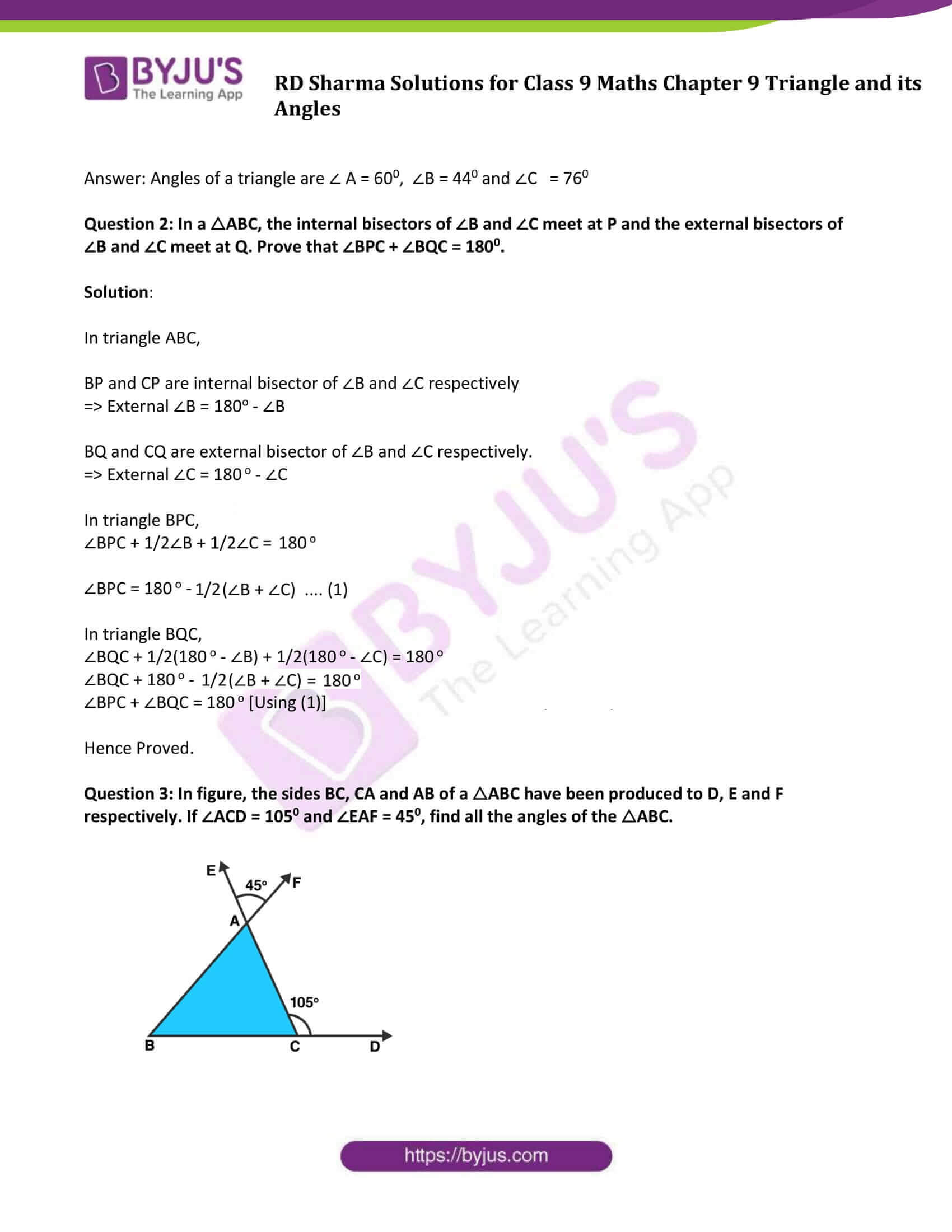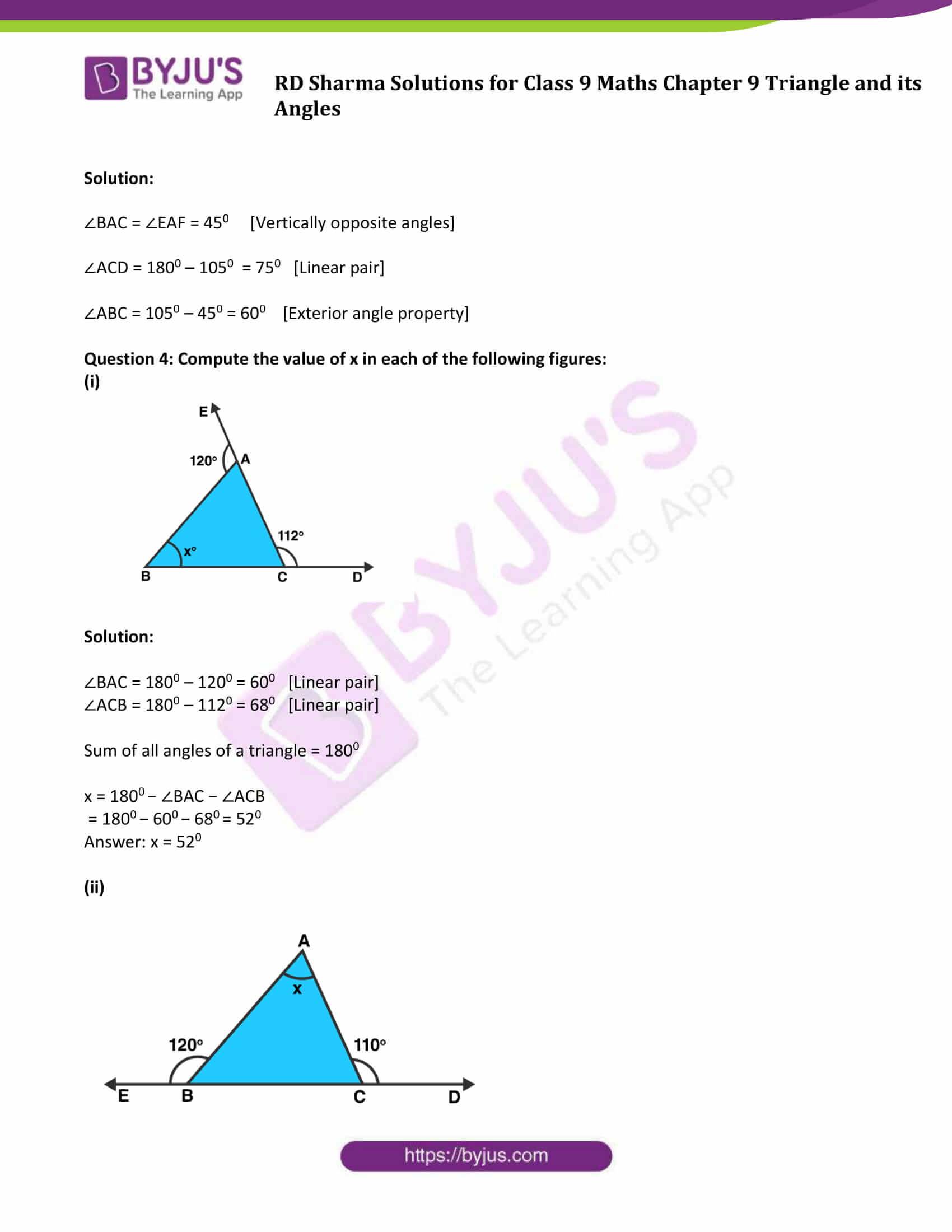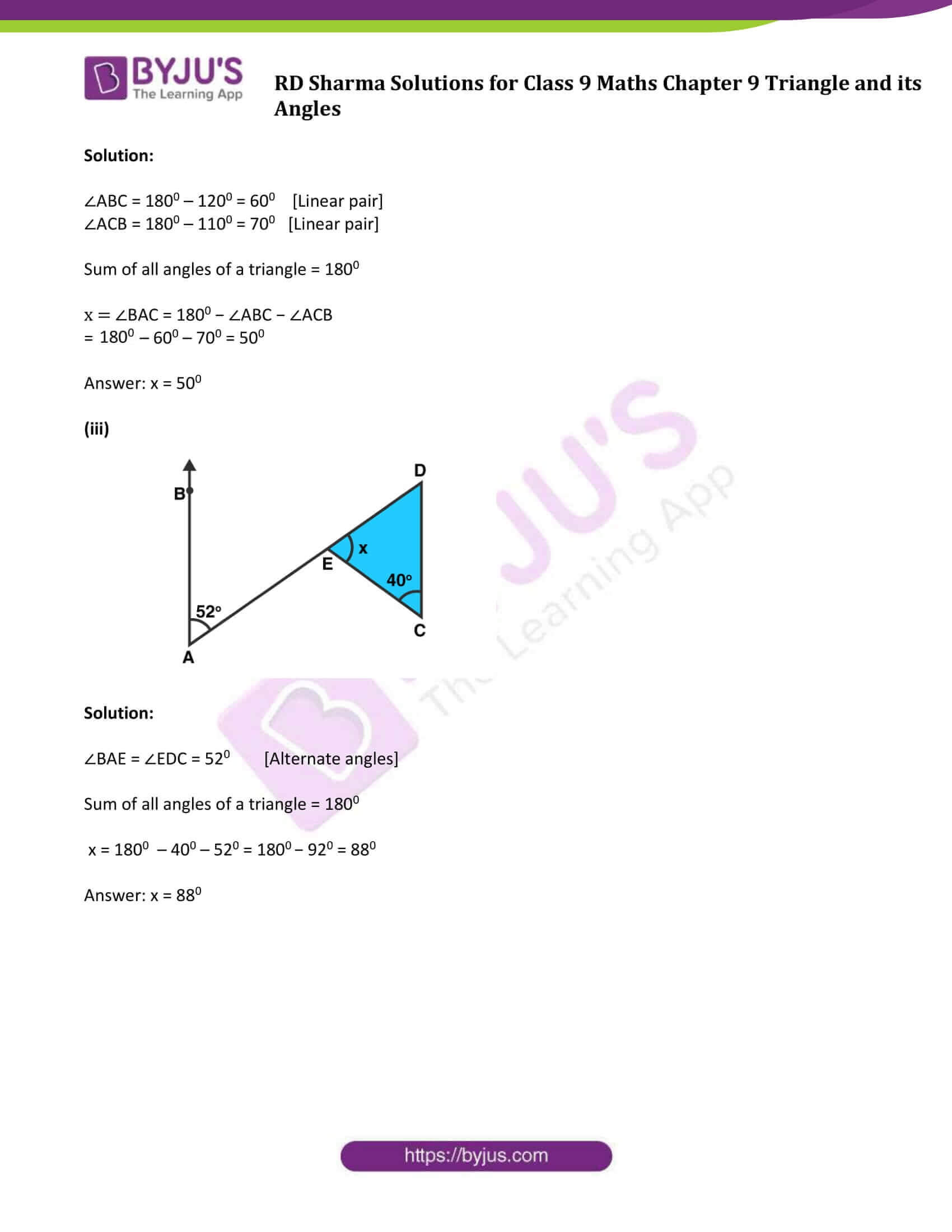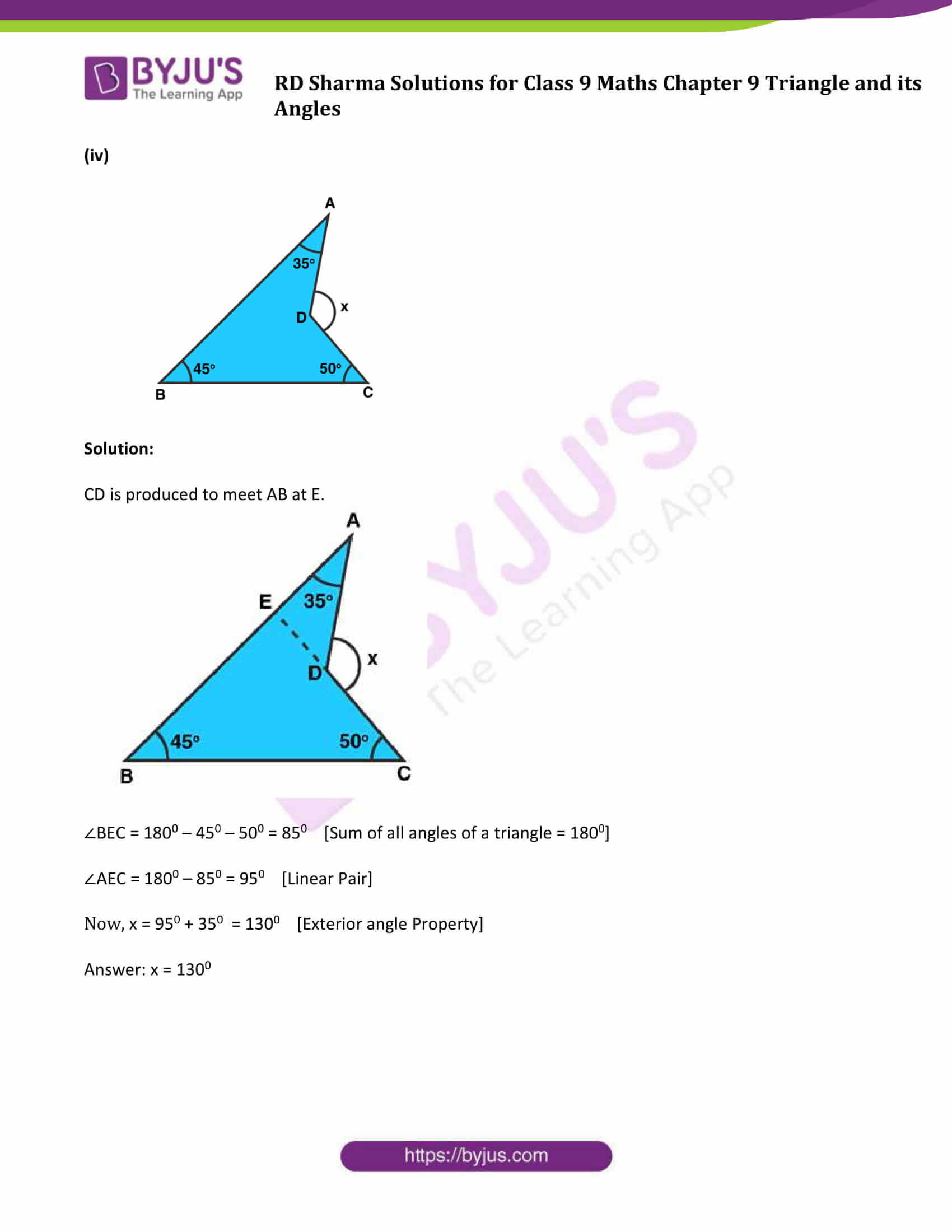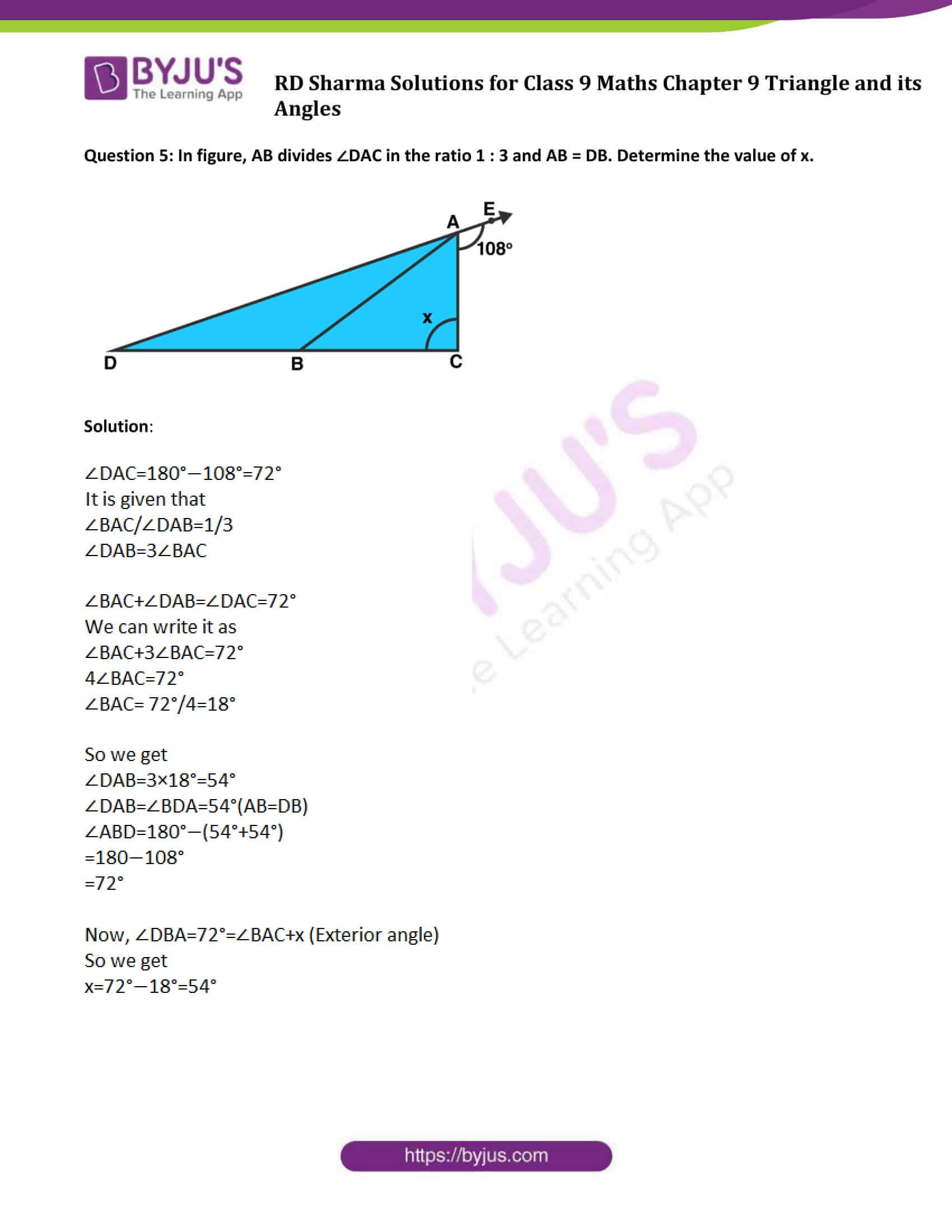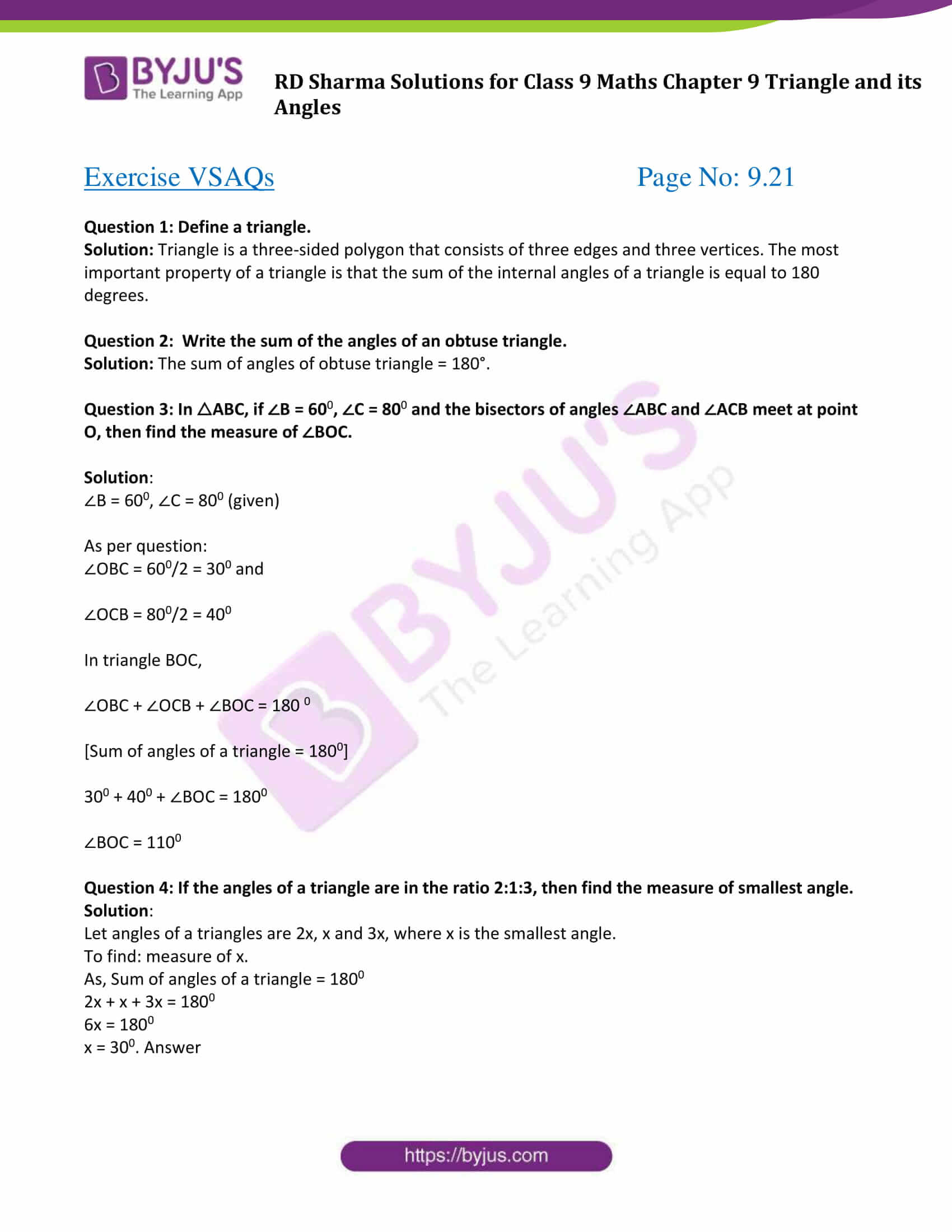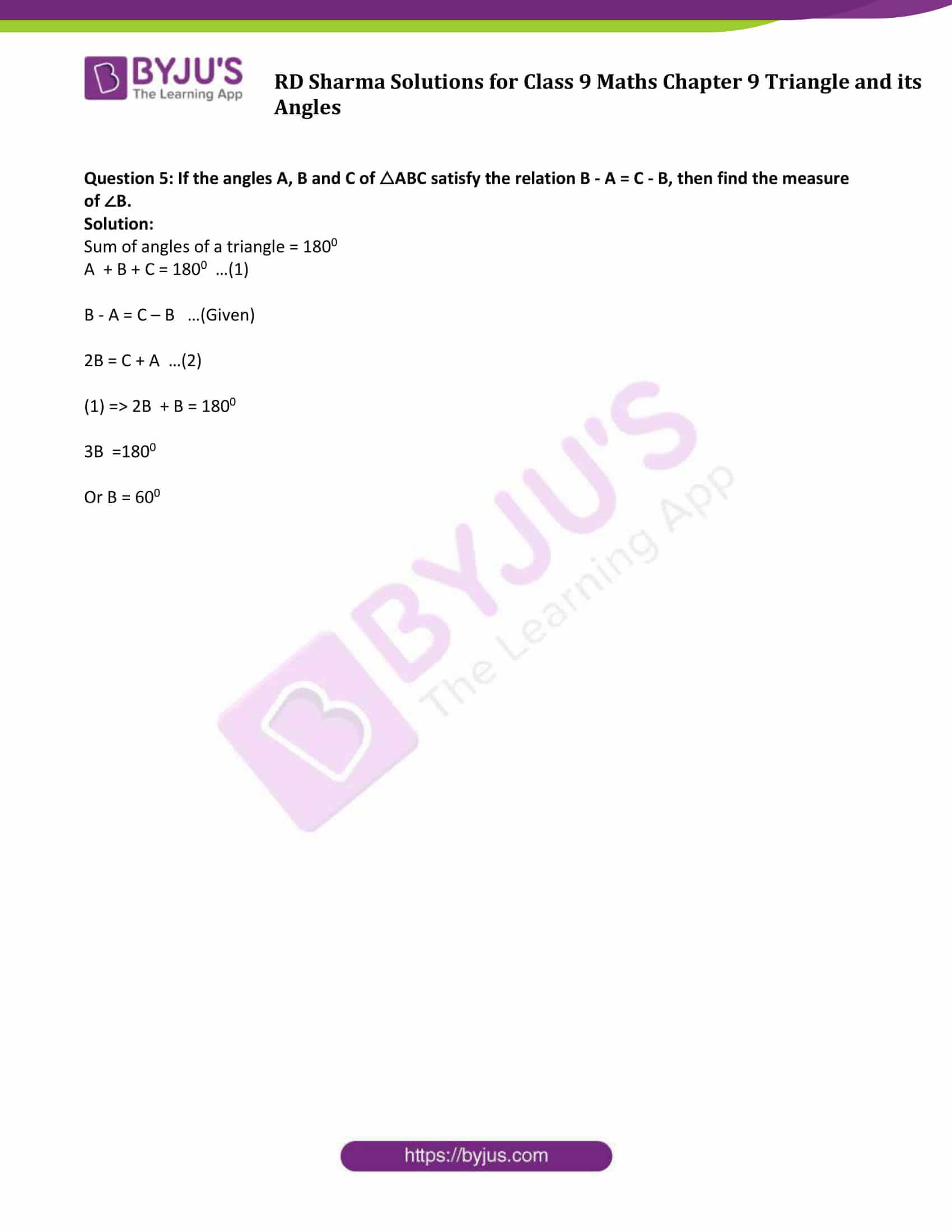### Access Answers to Maths RD Sharma Solutions for Class 9 Chapter 9 Triangle and its Angles

Exercise 9.1 Page No: 9.9

Question 1: In a ΔABC, if ∠A = 550, ∠B = 400, find ∠C.

Solution:

Given: ∠A = 550, ∠B = 400

We know, sum of all angles of a triangle is 1800

∠A + ∠B + ∠C = 1800

550 + 400 + ∠C=1800

950 + ∠C = 1800

∠C = 1800 − 950

∠C = 850

Question 2: If the angles of a triangle are in the ratio 1:2:3, determine three angles.

Solution:

Angles of a triangle are in the ratio 1:2:3 (Given)

Let the angles be x, 2x, 3x

Sum of all angles of triangles = 1800

x + 2x + 3x = 1800

6x = 1800

x = 1800/6

x = 300

x = 300

2x = 2(30)0 = 600

3x = 3(30) 0 = 900

Question 3: The angles of a triangle are (x − 40)0, (x − 20) 0 and (1/2 x − 10) 0. Find the value of x.

Solution:

The angles of a triangle are (x − 40)0, (x − 20) 0 and (1/2 x − 10) 0

Sum of all angles of triangle = 1800

(x − 40)0 + (x − 20) 0 + (1/2 x − 10) 0 = 1800

5/2 x – 700 = 1800

5/2 x = 1800 + 700

5x = 2(250) 0

x = 5000/5

x = 1000

Question 4: The angles of a triangle are arranged in ascending order of magnitude. If the difference between two consecutive angles is 100, find the three angles.

Solution:

The difference between two consecutive angles is 100 (given)

Let x, x + 100, x + 200 be the consecutive angles

x + x + 100 + x + 200 = 1800

3x + 300 = 1800

3x = 1800– 300

3x = 1500

or x = 500

Again,

x + 100 = 500 + 100 = 600

x+200 = 500 + 200 = 700

Answer: Three angles are 500,600 and 700.

Question 5: Two angles of a triangle are equal and the third angle is greater than each of those angles by 300. Determine all the angles of the triangle.

Solution:

Two angles of a triangle are equal and the third angle is greater than each of those angles by 300. (Given)

Let x, x, x + 300 be the angles of a triangle.

Sum of all angles in a triangle = 1800

x + x + x + 300 = 1800

3x + 300 = 1800

3x = 1500

or x = 500

And x + 300 = 500 + 300 = 800

Answer: Three angles are 500, 500 and 800.

Question 6: If one angle of a triangle is equal to the sum of the other two, show that the triangle is a right angle triangle.

Solution:

One angle of a triangle is equal to the sum of the other two angles (given)

To Prove: One of the angles is 900

Let x, y and z are three angles of a triangle, where

z = x + y …(1)

Sum of all angles of a triangle = 1800

x + y + z = 1800

z + z = 1800 (Using equation (1))

2z = 1800

z = 900 (Proved)

Therefore, triangle is a right angled triangle.

Exercise 9.2 Page No: 9.18

Question 1: The exterior angles, obtained on producing the base of a triangle both ways are 1040 and 1360. Find all the angles of the triangle.

Solution: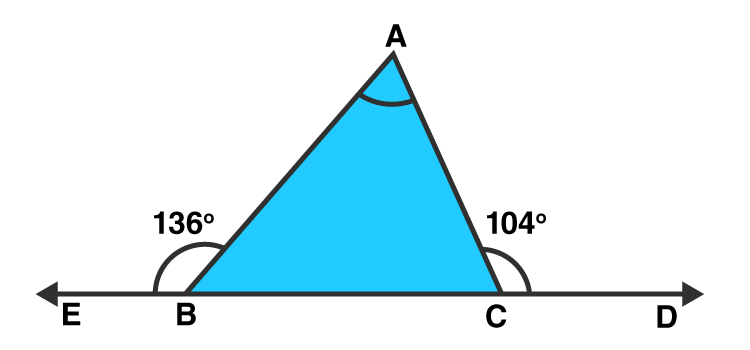∠ACD = ∠ABC + ∠BAC [Exterior angle property]

Find ∠ABC:

∠ABC + ∠ABE = 1800 [Linear pair]

∠ABC + 1360 = 1800

∠ABC = 440

Find ∠ACB:

∠ACB + ∠ACD = 1800 [Linear pair]

∠ACB + 1040 = 1800

∠ACB = 760

Now,

Sum of all angles of a triangle = 1800

∠A + 440 + 760 = 1800

∠A = 1800 − 440 −760

∠ A = 600

Answer: Angles of a triangle are ∠ A = 600, ∠B = 440 and ∠C = 760

Question 2: In a △ABC, the internal bisectors of ∠B and ∠C meet at P and the external bisectors of ∠B and ∠C meet at Q. Prove that ∠BPC + ∠BQC = 1800.

Solution:

In triangle ABC,

BP and CP are internal bisector of ∠B and ∠C respectively

=> External ∠B = 180o – ∠B

BQ and CQ are external bisector of ∠B and ∠C respectively.

=> External ∠C = 180 o – ∠C

In triangle BPC,

∠BPC + 1/2∠B + 1/2∠C = 180o

∠BPC = 180 o – 1/2(∠B + ∠C) …. (1)

In triangle BQC,

∠BQC + 1/2(180 o – ∠B) + 1/2(180 o – ∠C) = 180 o

∠BQC + 180 o – 1/2(∠B + ∠C) = 180 o

∠BPC + ∠BQC = 180 o [Using (1)]

Hence Proved.

Question 3: In figure, the sides BC, CA and AB of a △ABC have been produced to D, E and F respectively. If ∠ACD = 1050 and ∠EAF = 450, find all the angles of the △ABC.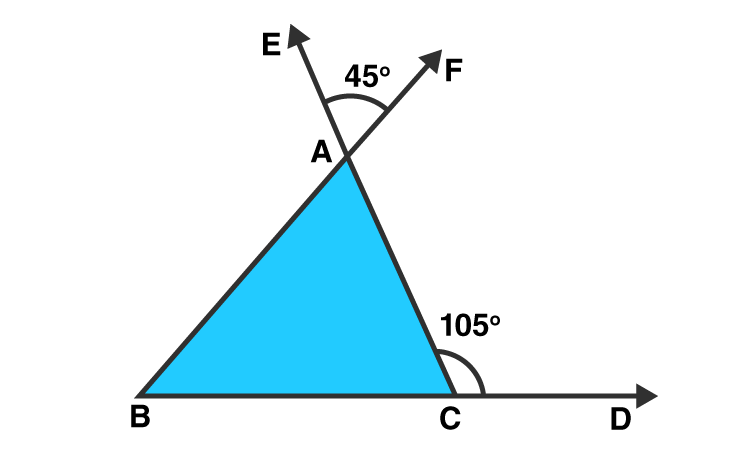Solution:

∠BAC = ∠EAF = 450 [Vertically opposite angles]

∠ACD = 1800 – 1050 = 750 [Linear pair]

∠ABC = 1050 – 450 = 600 [Exterior angle property]

Question 4: Compute the value of x in each of the following figures:

(i)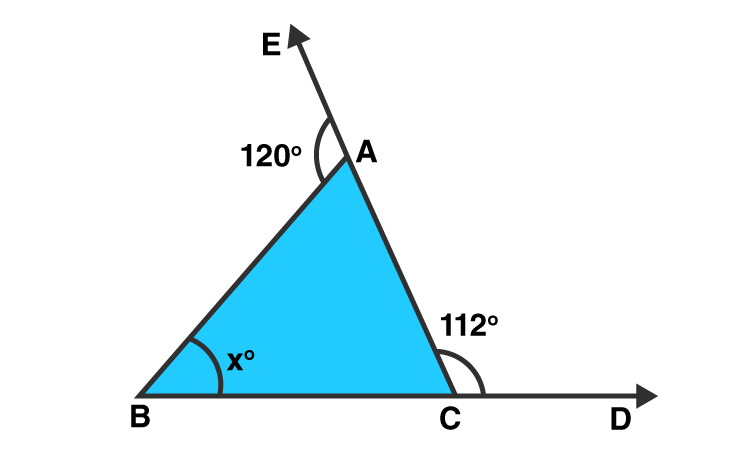Solution:

∠BAC = 1800 – 1200 = 600 [Linear pair]

∠ACB = 1800 – 1120 = 680 [Linear pair]

Sum of all angles of a triangle = 1800

x = 1800 − ∠BAC − ∠ACB

= 1800 − 600 − 680 = 520

(ii)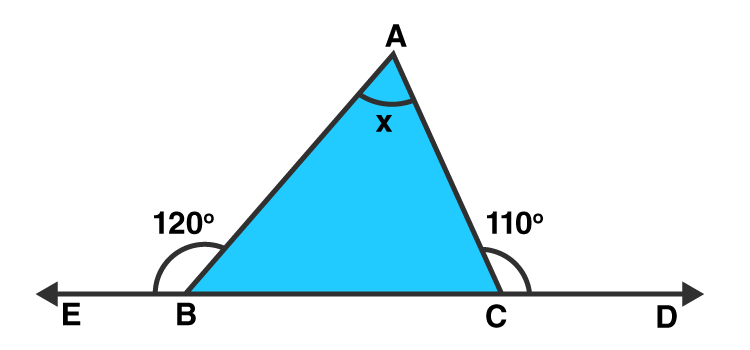Solution:

∠ABC = 1800 – 1200 = 600 [Linear pair]

∠ACB = 1800 – 1100 = 700 [Linear pair]

Sum of all angles of a triangle = 1800

x = ∠BAC = 1800 − ∠ABC − ∠ACB

= 1800 – 600 – 700 = 500

(iii)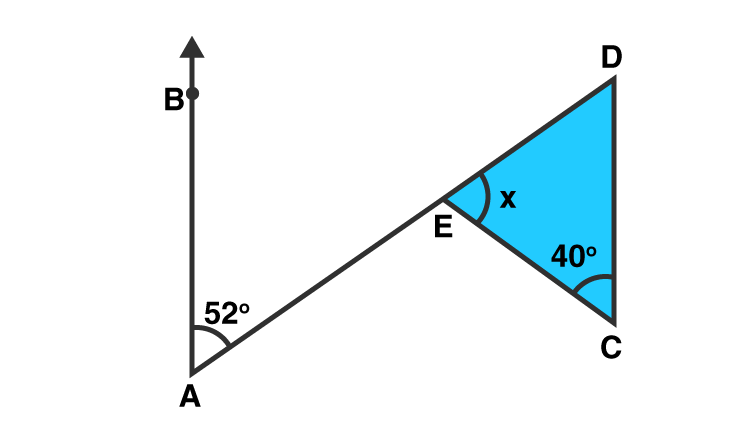Solution:

∠BAE = ∠EDC = 520 [Alternate angles]

Sum of all angles of a triangle = 1800

x = 1800 – 400 – 520 = 1800 − 920 = 880

(iv)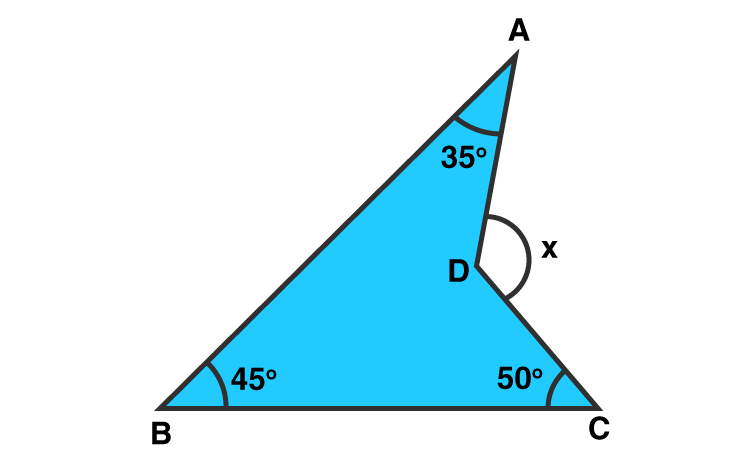Solution:

CD is produced to meet AB at E.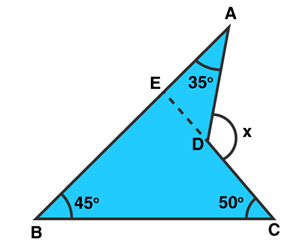∠BEC = 1800 – 450 – 500 = 850 [Sum of all angles of a triangle = 1800]

∠AEC = 1800 – 850 = 950 [Linear Pair]

Now, x = 950 + 350 = 1300 [Exterior angle Property]

Question 5: In figure, AB divides ∠DAC in the ratio 1 : 3 and AB = DB. Determine the value of x.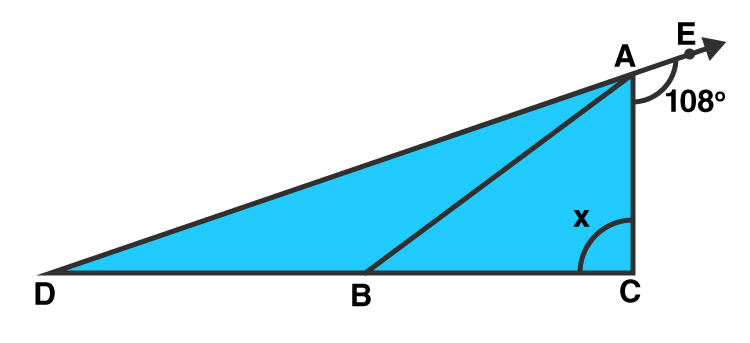Solution:

Now,
(Exterior angle)

Exercise VSAQs Page No: 9.21

Question 1: Define a triangle.

Solution: Triangle is a three-sided polygon that consists of three edges and three vertices. The most important property of a triangle is that the sum of the internal angles of a triangle is equal to 180 degrees.

Question 2: Write the sum of the angles of an obtuse triangle.

Solution: The sum of angles of obtuse triangle = 180°.

Question 3: In △ABC, if ∠B = 600, ∠C = 800 and the bisectors of angles ∠ABC and ∠ACB meet at point O, then find the measure of ∠BOC.

Solution:

∠B = 600, ∠C = 800 (given)

As per question:

∠OBC = 600/2 = 300 and

∠OCB = 800/2 = 400

In triangle BOC,

∠OBC + ∠OCB + ∠BOC = 180 0

[Sum of angles of a triangle = 1800]

300 + 400 + ∠BOC = 1800

∠BOC = 1100

Question 4: If the angles of a triangle are in the ratio 2:1:3, then find the measure of smallest angle.

Solution:

Let angles of a triangles are 2x, x and 3x, where x is the smallest angle.

To find: measure of x.

As, Sum of angles of a triangle = 1800

2x + x + 3x = 1800

6x = 1800

Question 5: If the angles A, B and C of △ABC satisfy the relation B – A = C – B, then find the measure of ∠B.

Solution:

Sum of angles of a triangle = 1800

A + B + C = 1800 …(1)

B – A = C – B …(Given)

2B = C + A …(2)

(1) => 2B + B = 1800

3B =1800

Or B = 600

## RD Sharma Solutions for Class 9 Maths Chapter 9 Triangle and Its Angles

In the 9th Chapter of Class 9 RD Sharma Solutions students will study important concepts listed below:

• Triangle Introduction
• Types of triangles
• Some important Theorems on triangles

Students can download all chapters solutions enlisted in RD Sharma Class 9 textbook and start practising to score good marks.

## Frequently Asked Questions on RD Sharma Solutions for Class 9 Maths Chapter 9

### Why should we download RD Sharma Solutions for Class 9 Maths Chapter 9 for better learning of concepts?

RD Sharma Solutions for Class 9 Maths is described in a precise manner by BYJU’S experts. The solutions are well structured in a simple and understandable language to improve the grasping abilities of students. In order to procure proficiency in Maths, students must practice RD Sharma Solutions as many times as possible. Students can make use of solutions in PDF to improve the skills required for solving problems effortlessly. The solutions can be accessed anytime and anywhere as per their needs.

### Why is RD Sharma Solutions for Class 9 Maths Chapter 9 essential to score high marks in board exams?

RD Sharma Solutions are the best source to understand complex concepts in an easy manner. Students who follow these solutions diligently clear their doubts quickly and also enhance problem-solving skills. RD Sharma Solutions for Class 9 Maths Chapter 9 are well formulated by experts at BYJU’S in step by step manner. This allows the students to boost confidence to solve any type of problem with ease and score good marks in examinations.

### Give a brief summary of concepts present in RD Sharma Solutions for Class 9 Maths Chapter 9.

The concepts discussed in RD Sharma Solutions for Class 9 Maths Chapter 9 are as follows:
Triangle Introduction
Types of triangles
Some important Theorems on triangles